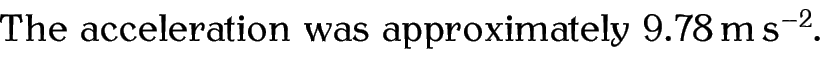Latest news 2021-09-06: new blog post "Legacy Documents and TeX Live Docker Images".

# 4.9 Formatting SI Units

If you need to typeset numbers and units then I strongly recommend that you use the siunitx package. This section just provides a brief introduction to that package. You will need to read the siunitx package documentation  if you want further details.

`\num`{<number>}

This command typesets <number>, adding appropriate spacing between number groups where necessary. It also adds a leading zero if omitted before the decimal point and identifies exponents. Note that the command recognises both “.” and “,” as the decimal marker. If you want one of these characters between number groups (instead of the default space) you can change the settings, but it's best to stick to the default settings unless instructed to do otherwise.

Example:

Out of `\num`{12890} experiments, `\num`{1289} of them had a mean squared error of `\num`{.346} and `\num`{128} of them had a mean squared error of `\num`{1.23e-6}.

Result:`\ang`{<angle>}

This command typesets an angle. The argument <angle> may be a single number or three (some possibly empty) values separated by semi-colons.

Example:

The result formed an arc from `\ang`{45} to `\ang`{60;2;3}.

Result:`\si`{<unit>}

This command typesets a unit. The <unit> can be formed from commands like `\metre`, `\gram`, `\second` or `\kilo`. (See the siunitx documentation  for the full list.)

Example:

The distance was measured in `\si`{`\kilo``\metre`} and the area in `\si`{`\kilo``\metre``\squared`}. The acceleration was given in `\si`{`\metre``\per``\square``\second`}.

Result:`\SI`{<number>}{<unit>}

This combines the functionality of `\num` and `\si` so that you can typeset both a number and a unit.

Example:

The acceleration was approximately `\SI`{9.78}{`\metre``\per``\square``\second`}.

Result:This book is also available as A4 PDF or 12.8cm x 9.6cm PDF or paperback (ISBN 978-1-909440-02-9).

© 2013 Dickimaw Books. "Dickimaw", "Dickimaw Books" and the Dickimaw parrot logo are trademarks. The Dickimaw parrot was painted by Magdalene Pritchett.